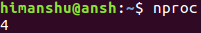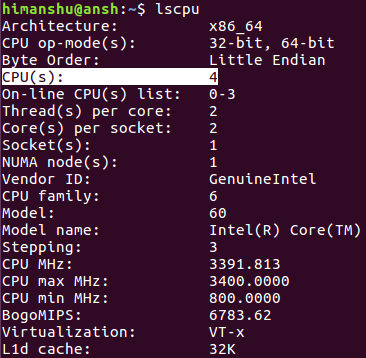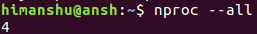# Linux nproc Command Tutorial for Beginners (with Examples)

Every process that's executed on a computer system requires CPU to do what it is expected to do. There may be times when your system's CPU is overloaded (due to the number or kind of processes running on the system), and for whatever reason, you want to know the number of available processing units for new processes. Well, there's a tool dubbed nproc that you can use to confirm this information.

In this tutorial, we will discuss the basics of nproc using some easy to understand examples. But before we do that, it's worth mentioning that all examples included in this article have been tested on Ubuntu 16.04 LTS.

## Linux nproc command

The nproc command basically displays in output the number of available processing units. Following is the tool's syntax:

``nproc [OPTION]...``

And here's how the utility's man page defines it:

``Print the number of processing units available to the current process, which may be less than the number of online processors``

Following are some Q&A-styled examples that will give you a good idea on how the nproc command works.

## Q1. How to use nproc?

This is very easy - all you have to do is to just run the 'nproc' command.

``nproc``

On my system, the tool produces the following output:So the output produced is '4'.

It's worth mentioning that this number does not represent the number of physical CPUs. The output of nproc corresponds to the CPUs field in the output of the lscpu command.And CPUs in itself is nothing but:

Threads per core X cores per socket X sockets

So in our case that comes out to be 2x2x1, which is equal to 4.

## Q2. How to make nproc print total installed processing units?

Instead of the number of available processing units, if you want nproc to display the total installed processing units, you can use the --all option.

``nproc --all``

For example, here's the option in action:So on my system, the total number of installed processing units is 4.

## Q3. How to make nproc exclude some processing units?

There exists a command line option --ignore which you can use to tell nproc that if possible, exclude a set number of processing units.

For example:

``nproc --ignore=2``

PS: In case you want to know more about the nproc command, you can use the --help and --version options.

## Conclusion

Clearly, nproc is not the kind of tool an average Linux command line user would require on day to day basis, but it's always good to know about such commands. However, if you are a system admin or someone whose work involves debugging Linux system related issues, the nproc command could be of great help. You can learn more about it by heading to its man page.view as pdf |print

Add comment